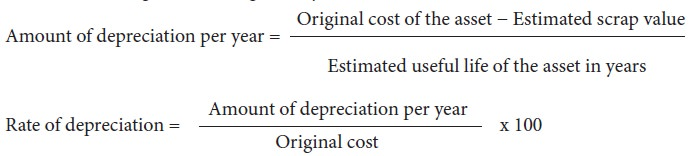Home | | Accountancy 11th std | Straight line method/ Fixed instalment method / Original cost method

# Straight line method/ Fixed instalment method / Original cost method

Under this method, a fixed percentage on the original cost of the asset is charged every year by way of depreciation.

Straight line method/ Fixed instalment method / Original cost method

Under this method, a fixed percentage on the original cost of the asset is charged every year by way of depreciation. Hence it is called original cost method. As the amount of depreciation remains equal in all years over the useful life of an asset it is also called as fixed instalment method. When the amount of depreciation charged over its life is plotted on a graph and the points are joined together, the graph will show a horizontal straight line. Hence, it is called straight line method.

This method is suitable for those assets the useful life of which can be estimated accurately and which do not require much expense on repairs and renewals.

Under this method, the following formulae are used for calculating the amount of depreciation and the rate of depreciation respectively:Tutorial note

·           In the year of purchase, if the period of use is less than a year, the amount of depreciation will be charged proportionately for the period for which the asset has been used in the business.

·           If depreciation is deducted from the cost of the asset at the end of useful life of the asset the amount left in the asset account will be equal to the scrap value if there is any scrap value or it will be zero if there is no scrap value.

Example

On 1.1.2012, a firm purchased a machine at a cost of Rs.  1,10,000. Its life was estimated to be 10 years with a scrap value of Rs.  10,000. The amount of depreciation to be charged at the end of each year is:When it is plotted on a graph for 5 years, it appears as follows:## Merits

Following are the merits of straight line method of depreciation:

### (a)  Simple and easy to understand

Computation of depreciation under this method is very simple and is easy to understand.

### (b)  Equality of depreciation burden

Under this method, equal amount of depreciation is debited to the profit and loss account each year. Hence, the burden of depreciation on the profit of each year is equal..

### (c)  Assets can be completely written off

Under this method, the book value of an asset can be reduced to zero if there is no scrap value or to the scrap value at the end of its useful life. Thus the asset account can be completely written off.

### (d)  Suitable for the assets having fixed working life

This method is appropriate for the fixed assets having certain fixed period of working life. In such cases, the estimation of useful life is easy and in turn it helps in easy determination of rate of depreciation.

## Limitations

Following are the limitations of straight line method of depreciation:

### (a)  Ignores the actual use of the asset

Under this method, a fixed amount of depreciation is provided on each asset by applying the predetermined rate of depreciation on its original cost. But, the actual use of the asset is not considered in computation of depreciation.

### (b)  Ignores the interest factor

This method does not take into account the loss of interest on the amount invested in the asset. That is, the amount would have earned interest, had it been invested outside the business is not considered.

### (c)  Total charge on the assets will be more when the asset becomes older

With the passage of time, the cost of maintenance of an asset goes up. Hence, the amount of depreciation and cost of maintenance put together is less in the initial period and goes up year after year. But, this method does not consider this.

### (d)  Difficulty in the determination of scrap value

It may be quite difficult to assess the true scrap value of the asset after a long period say 10 or 15 years after the date of its installation.

### Suitability

Straight line method of depreciation is suitable in case of fixed assets in respect of which useful life can be determined and maintenance and repair cost is the same throughout the life of the asset.

### Illustration 1

On 1.1.2017 a firm purchased a machine at a cost of Rs.  1,00,000. Its life was estimated to be 10 years with a scrap value of Rs.  10,000. Compute the amount of depreciation to be charged at the end of each year.

Solution### Illustration 2

A company has purchased a machinery for Rs.  1,80,000 and spent Rs.  10,000 for its installation. The estimated life of the machinery is 5 years with a residual value of Rs.  15,000. Find out the amount of depreciation to be provided every year.

Solution### Illustration 3

From the following information, calculate the amount of depreciation and rate of depreciation under straight line method.

Purchase price of machine       Rs.  2,00,000

Expenses to be capitalised      Rs. 50,000

Estimated residual value         Rs. 15,000

Expected useful life                 5 years

Solution### Illustration 4

Find out the rate of depreciation under straight line method from the following details:

Original cost of the asset             = Rs.  10,000

Estimated life of the asset            = 10 years

Estimated scrap value at the end = Rs.  2,000

SolutionTags : Example, Merits, Limitations, Example Illustration, Solution | Methods of providing depreciation | Accountancy , 11th Accountancy : Chapter 10 : Depreciation Accounting
Study Material, Lecturing Notes, Assignment, Reference, Wiki description explanation, brief detail
11th Accountancy : Chapter 10 : Depreciation Accounting : Straight line method/ Fixed instalment method / Original cost method | Example, Merits, Limitations, Example Illustration, Solution | Methods of providing depreciation | Accountancy